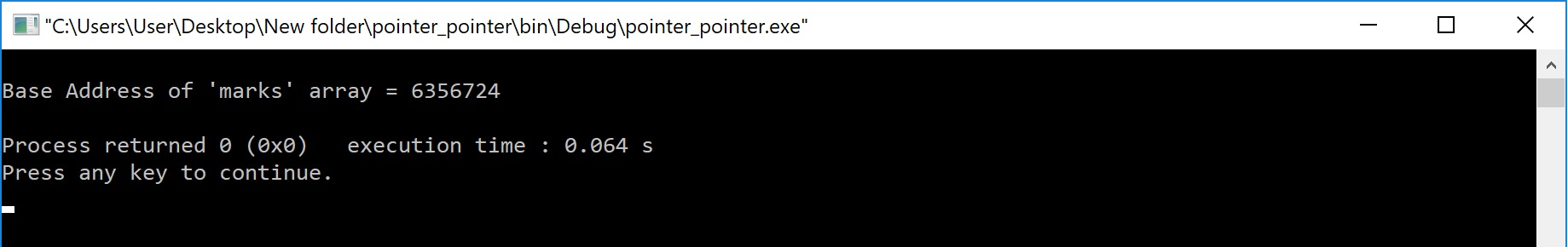The perfect place for easy learning...

×

# Pointers to Arrays in C

In the c programming language, when we declare an array the compiler allocate the required amount of memory and also creates a constant pointer with array name and stores the base address of that pointer in it. The address of the first element of an array is called as base address of that array.

The array name itself acts as a pointer to the first element of that array. Consider the following example of array declaration...

# Example Code

````int  marks ;`
```

For the above declaration, the compiler allocates 12 bytes of memory and the address of first memory location (i.e., marks) is stored in a constant pointer called marks. That means in the above example, marks is a pointer to marks.

# Example Program

``````#include<stdio.h>
#include<conio.h>

int main()
{
int marks = {89, 45, 58, 72, 90, 93} ;
int *ptr ;

clrscr() ;

ptr = marks ;
printf(“Base Address of 'marks' array = %u\n”, ptr) ;
return 0;
}```
```

Output:MOST IMPORTANT POINTS TO BE REMEMBERED

1. An array name is a constant pointer.
2. We can use the array name to access the address and value of all the elements of that array.
3. Since array name is a constant pointer we can't modify its value.

Consider the following example statements...

# Example Code

````ptr = marks + 2 ;`
```

Here, the pointer variable "ptr" is assigned with address of "marks" element.

# Example Code

````printf("Address of 'marks' = %u", marks+4) ;`
```

The above printf statement displays the address of element "marks".

# Example Code

``````printf("Value of 'marks' = %d", *marks) ;
printf("Value of 'marks' = %d", *(marks+3)) ;```
```

In the above two statements, first printf statement prints the value 89 (i.e., value of marks) and the second printf statement prints the value 72 (i.e., value of marks).

# Example Code

````marks++ ;`
```

The above statement generates compilation error because the array name acts as a constant pointer. So we can't change its value.

In the above example program, the array name marks can be used as follows...

marks is same as &marks
marks + 1 is same as &marks
marks + 2 is same as &marks
marks + 3 is same as &marks
marks + 4 is same as &marks
marks + 5 is same as &marks
*marks is same as marks
*(marks + 1) is same as marks
*(marks + 2) is same as marks
*(marks + 3) is same as marks
*(marks + 4) is same as marks
*(marks + 5) is same as marks

## Pointers to Multi Dimensional Array

In case of multi dimensional array also the array name acts as a constant pointer to the base address of that array. For example, we declare an array as follows...

# Example Code

````int  marks ;`
```

In the above example declaration, the array name marks acts as constant pointer to the base address (address of marks) of that array.
In the above example of two dimensional array, the element marks is accessed as *(*(marks + 1) + 2).